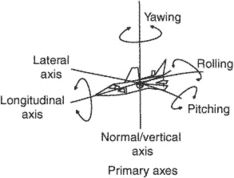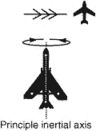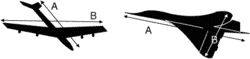# principal axis

(redirected from Principle axis)
Also found in: Dictionary, Thesaurus, Medical.
Related to Principle axis: centre of curvature

## principal axis

[′prin·sə·pəl ′ak·səs]
(crystallography)
The longest axis in a crystal.
(engineering acoustics)
A reference direction for angular coordinates used in describing the directional characteristics of a transducer; it is usually an axis of structural symmetry or the direction of maximum response.
(mathematics)
One of a set of perpendicular axes such that a quadratic function can be written as a sum of squares of coordinates referred to these axes.
For a conic, a straight line that passes through the midpoints of all the chords perpendicular to it.
For a quadric surface, the intersection of two principal planes.
(mechanics)
One of three perpendicular axes in a rigid body such that the products of inertia about any two of them vanish.
(optics)
McGraw-Hill Dictionary of Scientific & Technical Terms, 6E, Copyright © 2003 by The McGraw-Hill Companies, Inc.

## principal axisPrimary axesIn the illustration on the left, line A is the principal axis while in the illustration on the right, line B is the principal axis. The moment of inertia is maximum around these axes in these two cases.
i. The lateral, vertical, and longitudinal axes.
ii. The axis of relative wind.
iii. The principal inertial axis: that line passing through the center of gravity in a plane of symmetry about which a long, slender body tends to rotate when rolling.
iv. A rectilinear axis in the plane of a cross section of a structural member about which the moment of inertia is the maximum.
An Illustrated Dictionary of Aviation Copyright © 2005 by The McGraw-Hill Companies, Inc. All rights reserved
Site: Follow: Share:
Open / Close